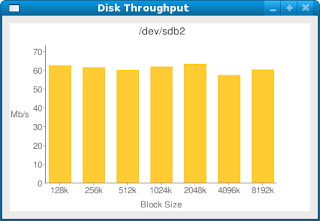# Not your Grandfather's dd Utility!

A recent article in Red Hat Magazine by Noah Gift and Grig Gheorghiu called “This isn’t your grandpappy’d dd command demonstrated how to use Python, the dd utility and the Google Chart API to produce a bar chart showing throughput at different block sizes. However the output from the Python script was not the actual graph but a URL which you then had to paste into a Web browser to view the resulting chart.

I though this script would be useful but did not want to have to cut and paste a URL into a Web browser so I decided to eliminate that step.

This Python script is loosely based on their script but uses the Python urllib libraries to connect to Google Charts to generate a PNG image file which is subsequently displayed using pyGTK+ routines.

```#!/usr/bin/env python

import sys
import os
import commands
import re
from optparse import OptionParser
import urllib
import urllib2
import pygtk
pygtk.require('2.0')
import gtk

class DisplayGraph:

def delete_event(self, widget, event, data=None):
return False

def destroy(self, widget, data=None):
gtk.main_quit()

def __init__(self):
self.window = gtk.Window(gtk.WINDOW_TOPLEVEL)
self.window.connect("delete_event", self.delete_event)
self.window.connect("destroy", self.destroy)
self.window.set_border_width(10)
self.window.set_position(gtk.WIN_POS_CENTER)
self.window.set_title("Disk Throughput")

pixbuf = gtk.gdk.pixbuf_new_from_file("/tmp/dd.png")
os.remove("/tmp/dd.png")

self.image = gtk.Image()
self.image.set_from_pixbuf(pixbuf)
self.image.show()
self.window.show()

def main(self):
gtk.main()

def __init__(self):
self.gchart_type = "cht=bvs"
self.gchart_title = "&chtt="
self.gchart_data = "&chd=t:"
self.gchart_labels = "&chxl=0:|"
self.gchart_size = "&chs=400x250"
self.gchart_axis_labels = "&chxt=x,y,x,y"
self.gchart_axis_position = "&chxp=2,50|3,50"
self.gchart_bar_settings = "&chbh=30,15"

def title(self,title):
self.gchart_title = self.gchart_title + title

def write(self, data, labels, max_t):
self.gchart_data = self.gchart_data + data.rstrip(',')
self.gchart_labels = self.gchart_labels  \
+ labels + "2:|Block%20Size|3:|Mb/s"
self.gchart_axis_range = "&chxr=1,0," + str(max_t+10.0)
self.gchart_scaling = "&chds=0," + str(max_t+10.0)
self.gchart_url += self.gchart_type \
+ self.gchart_title + self.gchart_size
self.gchart_url += self.gchart_bar_settings \
+ self.gchart_data + self.gchart_labels
self.gchart_url += self.gchart_axis_labels  \
+ self.gchart_axis_position
self.gchart_url += self.gchart_axis_range \
+ self.gchart_scaling

opener = urllib2.urlopen(self.gchart_url)
'with a content-type of %s' \

def get_disk_throughput(device, blocksize):
blocksize = str(blocksize) + 'k'
cmd = "dd if=/dev/zero of=%s bs=%s" % (device,blocksize)
output = commands.getoutput(cmd)

throughput = 0
unit = ""
for line in output.split('n'):
s = re.search(' copied,.*, (\S+) (\S+)\$', line)
if s:
throughput = s.group(1)
unit = s.group(2)
break
return (throughput, unit)

if __name__ == "__main__":
usage = "Usage: %prog options"
parser = OptionParser(usage=usage)
help="Device to use. Disk data will be overwritten!")
(options, args) = parser.parse_args()
device = options.device
if not device:
parser.print_help()
sys.exit(1)

max_t = 0.0
# block sizes to test dd write
blocksizes = [128, 256, 512, 1024, 2048, 4096, 8192]
data=""
labels=""
for blocksize in blocksizes:
(t, u) = get_disk_throughput(device, blocksize)
if float(t) > max_t:
max_t = float(t)
data += str(t) + ","
labels += str(blocksize) + "k" + "|"

chart.title(device)
chart.write(data, labels, max_t)

graph = DisplayGraph()
graph.main()
```

Here is a snapshot of a typical graph producedBTW, this script was developed and tested on Fedora 9. Enjoy!